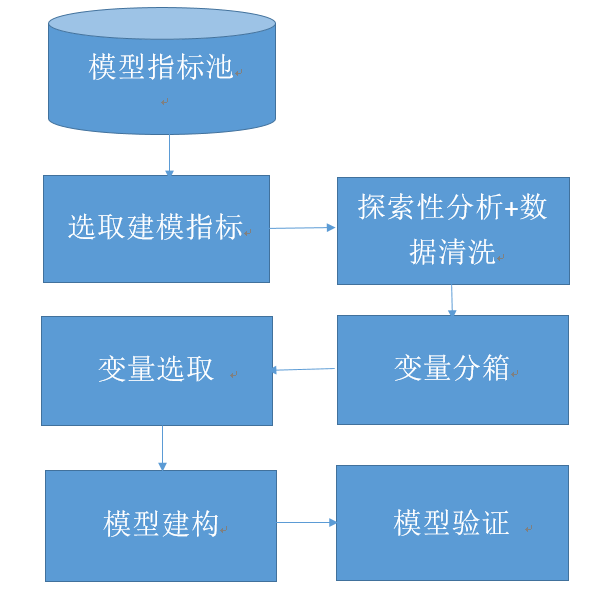# 【R语言 评分模型】R语言建立信用评分模型

1、数据源：

3、建模过程4、完整版（源代码）：

rm(list=ls())
gc()
library(caret)
library(smbinning)
library(ggplot2)
library(woe)

train <-createDataPartition(y=german_credit$Creditability,p=0.75,list=FALSE) train2 <- german_credit[train, ] test2 <- german_credit[-train, ] #Explore data distribution ggplot(german_credit, aes(x = Duration,y = ..count..,)) + geom_histogram(fill = "blue", colour = "grey60", size = 0.2, alpha = 0.2,binwidth = 5) ggplot(german_credit, aes(x = CreditAmount,y = ..count..,)) + geom_histogram(fill = "blue", colour = "grey60", size = 0.2, alpha = 0.2,binwidth = 1000) ggplot(german_credit, aes(x = Age,y = ..count..,)) + geom_histogram(fill = "blue", colour = "grey60", size = 0.2, alpha = 0.2,binwidth = 5) ggplot(german_credit, aes(x =Creditability,y = ..count..,)) + geom_histogram(fill = "blue", colour = "grey60" , alpha = 0.2,binwidth = 0.5) #Optimal Binning Durationresult=smbinning(df=train2,y="Creditability",x="Duration",p=0.05) CreditAmountresult=smbinning(df=train2,y="Creditability",x="CreditAmount",p=0.05) Ageresult=smbinning(df=train2,y="Creditability",x="Age",p=0.05) smbinning.plot(CreditAmountresult,option="WoE",sub="CreditAmount") smbinning.plot(Durationresult,option="WoE",sub="Duration") smbinning.plot(Ageresult,option="WoE",sub="Age") #Univariate Analysis AccountBalancewoe=woe(train2, "AccountBalance",Continuous = F, "Creditability",C_Bin = 4,Good = "1",Bad = "0") ggplot(AccountBalancewoe, aes(x = BIN, y = -WOE)) + geom_bar(stat = "identity",fill = "blue", colour = "grey60",size = 0.2, alpha = 0.2)+labs(title = "AccountBalance") ValueSavingswoe=woe(train2, "ValueSavings",Continuous = F, "Creditability",C_Bin = 5,Good = "1",Bad = "0") ggplot(ValueSavingswoe, aes(x = BIN, y = -WOE)) + geom_bar(stat = "identity",fill = "blue", colour = "grey60",size = 0.2, alpha = 0.2)+labs(title = "ValueSavings") Lengthofcurrentemploymentwoe=woe(train2, "Lengthofcurrentemployment",Continuous = F, "Creditability",C_Bin = 4,Good = "1",Bad = "0") ggplot(Lengthofcurrentemploymentwoe, aes(x = BIN, y = -WOE)) + geom_bar(stat = "identity",fill = "blue", colour = "grey60",size = 0.2, alpha = 0.2)+labs(title = "Lengthofcurrentemployment") #combine some bins for(i in 1:750){ if(train2$ValueSavings[i]==1){train2$ValueSavings[i]=2} } for(i in 1:750){ if(train2$Lengthofcurrentemployment[i]==5){train2$Lengthofcurrentemployment[i]=4} } library(corrplot) #correlation analysis cor1<-cor(train) corrplot(cor1,tl.cex = 0.5) #Infomation Value calculation for(i in 1:1000){ if(german_credit$Duration[i]<=11){german_credit$Duration[i]=1} else if(german_credit$Duration[i]<=30){german_credit$Duration[i]=2} else{german_credit$Duration[i]=3}
}

for(i in 1:1000){
if(german_credit$Age[i]<=25){german_credit$Age[i]=1}
else{german_credit$Age[i]=2} } for(i in 1:1000){ if(german_credit$CreditAmount[i]<=6742){german_credit$CreditAmount[i]=1} else{german_credit$CreditAmount[i]=2}
}

for(i in 1:1000){
if(german_credit$ValueSavings[i]==1){german_credit$ValueSavings[i]=2}
}

for(i in 1:1000){
if(german_credit$Lengthofcurrentemployment[i]==5){german_credit$Lengthofcurrentemployment[i]=4}
}

AccountBalancewoe=woe(train2, "AccountBalance",Continuous = F, "Creditability",C_Bin = 4,Good = "1",Bad = "0")
Durationwoe=woe(train2, "Duration",Continuous = F, "Creditability",C_Bin = 2,Good = "1",Bad = "0")
PaymentStatusofPreviousCreditwoe=woe(train2, "PaymentStatusofPreviousCredit",Continuous = F, "Creditability",C_Bin = 4,Good = "1",Bad = "0")
Purposewoe = woe(train2, "Purpose",Continuous = F, "Creditability",C_Bin = 11,Good = "1",Bad = "0")
CreditAmountwoe= woe(train2, "CreditAmount",Continuous = F, "Creditability",C_Bin = 2,Good = "1",Bad = "0")
ValueSavingswoe =woe(train2, "ValueSavings",Continuous = F, "Creditability",C_Bin = 4,Good = "1",Bad = "0")
Lengthofcurrentemploymentwoe=woe(train2, "Lengthofcurrentemployment",Continuous = F, "Creditability",C_Bin = 4,Good = "1",Bad = "0")
Instalmentpercenwoet=woe(train2, "Instalmentpercent",Continuous = F, "Creditability",C_Bin = 4,Good = "1",Bad = "0")
Sex.Marital.Statuswoe=woe(train2, "Sex.Marital.Status",Continuous = F, "Creditability",C_Bin = 4,Good = "1",Bad = "0")
Guarantorswoe=woe(train2, "Guarantors",Continuous = F, "Creditability",C_Bin = 2,Good = "1",Bad = "0")
Mostvaluableavailableassetwoe=woe(train2, "Mostvaluableavailableasset",Continuous = F, "Creditability",C_Bin = 4,Good = "1",Bad = "0")
Agewoe=woe(train2, "Age",Continuous = F, "Creditability",C_Bin = 2,Good = "1",Bad = "0")
ConcurrentCreditswoe=woe(train2, "ConcurrentCredits",Continuous = F, "Creditability",C_Bin = 3,Good = "1",Bad = "0")
Typeofapartmentwoe=woe(train2, "Typeofapartment",Continuous = F, "Creditability",C_Bin = 3,Good = "1",Bad = "0")
NoofCreditatthisBankwoe=woe(train2, "NoofCreditatthisBank",Continuous = F, "Creditability",C_Bin = 3,Good = "1",Bad = "0")
Occupationwoe=woe(train2, "Occupation",Continuous = F, "Creditability",C_Bin = 4,Good = "1",Bad = "0")
Noofdependentswoe=woe(train2, "Noofdependents",Continuous = F, "Creditability",C_Bin = 2,Good = "1",Bad = "0")
Telephonewoe=woe(train2, "Telephone",Continuous = F, "Creditability",C_Bin = 2,Good = "1",Bad = "0")
ForeignWorkerwoe=woe(train2, "ForeignWorker",Continuous = F, "Creditability",C_Bin = 2,Good = "1",Bad = "0")

va = c("AccountBalance",  "Duration",   "PaymentStatusofPreviousCredit",    "Purpose",  "CreditAmount", "ValueSavings", "Lengthofcurrentemployment",    "Instalmentpercent","Sex.Marital.Status","Guarantors","DurationinCurrentaddress",   "Mostvaluableavailableasset",   "Age","ConcurrentCredits","Typeofapartment",    "NoofCreditatthisBank","Occupation","Noofdependents",   "Telephone" ,"ForeignWorker")
iv=c(sum(AccountBalancewoe$IV),sum(Durationwoe$IV),sum(PaymentStatusofPreviousCreditwoe$IV),sum(Purposewoe$IV),sum(CreditAmountwoe$IV),sum(ValueSavingswoe$IV),sum(Lengthofcurrentemploymentwoe$IV) ,sum(Instalmentpercenwoet$IV) ,sum(Sex.Marital.Statuswoe$IV) ,sum(Guarantorswoe$IV)  ,sum(DurationinCurrentaddresswoe$IV) ,sum(Mostvaluableavailableassetwoe$IV),sum(Agewoe$IV),sum(ConcurrentCreditswoe$IV),sum(Typeofapartmentwoe$IV),sum(NoofCreditatthisBankwoe$IV),sum(Occupationwoe$IV), sum(Noofdependentswoe$IV), sum(Telephonewoe$IV),sum(ForeignWorkerwoe$IV))
infovalue = data.frame(va,iv)
ggplot(infovalue, aes(x = va, y = iv)) + geom_bar(stat = "identity",fill = "blue", colour = "grey60",size = 0.2, alpha = 0.2)+labs(title = "Information value")+ theme(axis.text.x=element_text(angle=90,colour="black",size=10));

#WoE transformation
german_credit$DurationinCurrentaddress=NULL german_credit$Guarantors=NULL
german_credit$Instalmentpercent=NULL german_credit$NoofCreditatthisBank=NULL
german_credit$Occupation=NULL german_credit$Noofdependents=NULL
german_credit$Telephone=NULL AccountBalancewoe=woe(train2, "AccountBalance",Continuous = F, "Creditability",C_Bin = 4,Good = "1",Bad = "0") Durationwoe=woe(train2, "Duration",Continuous = F, "Creditability",C_Bin = 2,Good = "1",Bad = "0") PaymentStatusofPreviousCreditwoe=woe(train2, "PaymentStatusofPreviousCredit",Continuous = F, "Creditability",C_Bin = 4,Good = "1",Bad = "0") Purposewoe = woe(train2, "Purpose",Continuous = F, "Creditability",C_Bin = 11,Good = "1",Bad = "0") CreditAmountwoe= woe(train2, "CreditAmount",Continuous = F, "Creditability",C_Bin = 2,Good = "1",Bad = "0") ValueSavingswoe =woe(train2, "ValueSavings",Continuous = F, "Creditability",C_Bin = 4,Good = "1",Bad = "0") Lengthofcurrentemploymentwoe=woe(train2, "Lengthofcurrentemployment",Continuous = F, "Creditability",C_Bin = 4,Good = "1",Bad = "0") Sex.Marital.Statuswoe=woe(train2, "Sex.Marital.Status",Continuous = F, "Creditability",C_Bin = 4,Good = "1",Bad = "0") Mostvaluableavailableassetwoe=woe(train2, "Mostvaluableavailableasset",Continuous = F, "Creditability",C_Bin = 4,Good = "1",Bad = "0") Agewoe=woe(train2, "Age",Continuous = F, "Creditability",C_Bin = 2,Good = "1",Bad = "0") ConcurrentCreditswoe=woe(train2, "ConcurrentCredits",Continuous = F, "Creditability",C_Bin = 3,Good = "1",Bad = "0") Typeofapartmentwoe=woe(train2, "Typeofapartment",Continuous = F, "Creditability",C_Bin = 3,Good = "1",Bad = "0") ForeignWorkerwoe=woe(train2, "ForeignWorker",Continuous = F, "Creditability",C_Bin = 2,Good = "1",Bad = "0") for(i in 1:1000){ for(s in 1:4){ if(german_credit$AccountBalance[i]==s){
german_credit$AccountBalance[i]=-AccountBalancewoe$WOE[s]
}
}

for(s in 1:3){
if(german_credit$Duration[i]==s){ german_credit$Duration[i]=-Durationwoe$WOE[s] } } for(s in 0:4){ if(german_credit$PaymentStatusofPreviousCredit[i]==s){
german_credit$PaymentStatusofPreviousCredit[i]=-PaymentStatusofPreviousCreditwoe$WOE[s+1]
}
}

for(s in 0:10){
if(s<=6){
if(german_credit$Purpose[i]==s){ german_credit$Purpose[i]=-Purposewoe$WOE[s+1] } }else{ if(german_credit$Purpose[i]==s){
german_credit$Purpose[i]=-Purposewoe$WOE[s]
}
}
}

for(s in 1:2){
if(german_credit$CreditAmount[i]==s){ german_credit$CreditAmount[i]=-CreditAmountwoe$WOE[s] } } for(s in 2:5){ if(german_credit$ValueSavings[i]==s){
german_credit$ValueSavings[i]=-ValueSavingswoe$WOE[s-1]
}
}

for(s in 1:5){
if(german_credit$Lengthofcurrentemployment[i]==s){ german_credit$Lengthofcurrentemployment[i]=-Lengthofcurrentemploymentwoe$WOE[s] } } for(s in 1:5){ if(german_credit$Sex.Marital.Status[i]==s){
german_credit$Sex.Marital.Status[i]=-Sex.Marital.Statuswoe$WOE[s]
}
}

for(s in 1:4){
if(german_credit$Mostvaluableavailableasset[i]==s){ german_credit$Mostvaluableavailableasset[i]=-Mostvaluableavailableassetwoe$WOE[s] } } for(s in 1:2){ if(german_credit$Age[i]==s){
german_credit$Age[i]=-Agewoe$WOE[s]
}
}

for(s in 1:5){
if(german_credit$ConcurrentCredits[i]==s){ german_credit$ConcurrentCredits[i]=-ConcurrentCreditswoe$WOE[s] } } for(s in 1:5){ if(german_credit$Typeofapartment[i]==s){
german_credit$Typeofapartment[i]=-Typeofapartmentwoe$WOE[s]
}
}

for(s in 1:2){
if(german_credit$ForeignWorker[i]==s){ german_credit$ForeignWorker[i]=-ForeignWorkerwoe$WOE[s] } } } #Stepwise regression & Logistic model buiding fit<-glm(Creditability~ AccountBalance + Duration +PaymentStatusofPreviousCredit +Purpose + CreditAmount + ValueSavings + Lengthofcurrentemployment +Sex.Marital.Status+ Mostvaluableavailableasset + Age + ConcurrentCredits + Typeofapartment + ForeignWorker,train2,family = "binomial") backwards = step(fit) summary(backwards) fit2<-glm(Creditability~ AccountBalance + Duration +PaymentStatusofPreviousCredit +Purpose + CreditAmount + ValueSavings + Lengthofcurrentemployment + Age + ConcurrentCredits + ForeignWorker,train2,family = "binomial") summary(fit2) fit3<-glm(Creditability~ AccountBalance + Duration +PaymentStatusofPreviousCredit +Purpose + CreditAmount + ValueSavings + Lengthofcurrentemployment + Age + ForeignWorker,train2,family = "binomial") summary(fit3) #VIF testing library(car) vif(fit3, digits =3) #producing confusion matrix prediction <- predict(fit3,newdata=test2) for (i in 1:250) { if(prediction[i]>0.99){ prediction[i]=1} else {prediction[i]=0} } confusionMatrix(prediction, test2$Creditability)

coe = (fit3$coefficients) p <- 20/log(2) q <- 600-20*log(2.5)/log(2) base <- q + p*as.numeric(coe) #score for AccountBalance AccountBalanceSCORE = p*as.numeric(coe)*AccountBalancewoe$woe*-1

p*as.numeric(coe)*AccountBalancewoe$WOE*-1 p*as.numeric(coe)*AccountBalancewoe$WOE*-1
p*as.numeric(coe)*AccountBalancewoe$WOE*-1 p*as.numeric(coe)*AccountBalancewoe$WOE*-1

#score for Duration
p*as.numeric(coe)*Durationwoe$WOE*-1 p*as.numeric(coe)*Durationwoe$WOE*-1
p*as.numeric(coe)*Durationwoe$WOE*-1 #score for PaymentStatusofPreviousCredit p*as.numeric(coe)*PaymentStatusofPreviousCreditwoe$WOE*-1
p*as.numeric(coe)*PaymentStatusofPreviousCreditwoe$WOE*-1 p*as.numeric(coe)*PaymentStatusofPreviousCreditwoe$WOE*-1
p*as.numeric(coe)*PaymentStatusofPreviousCreditwoe$WOE*-1 p*as.numeric(coe)*PaymentStatusofPreviousCreditwoe$WOE*-1

#score for purpose
for(i in 1:10){
print(p*as.numeric(coe)*Purposewoe$WOE[i])*-1 } p*as.numeric(coe)*CreditAmountwoe$WOE*-1
p*as.numeric(coe)*CreditAmountwoe$WOE*-1 p*as.numeric(coe)*ValueSavingswoe$WOE*-1
p*as.numeric(coe)*ValueSavingswoe$WOE*-1 p*as.numeric(coe)*ValueSavingswoe$WOE*-1
p*as.numeric(coe)*ValueSavingswoe$WOE*-1 p*as.numeric(coe)*Lengthofcurrentemploymentwoe$WOE*-1
p*as.numeric(coe)*Lengthofcurrentemploymentwoe$WOE*-1 p*as.numeric(coe)*Lengthofcurrentemploymentwoe$WOE*-1
p*as.numeric(coe)*Lengthofcurrentemploymentwoe$WOE*-1 p*as.numeric(coe)*Agewoe$WOE*-1
p*as.numeric(coe)*Agewoe$WOE*-1 p*as.numeric(coe)*ForeignWorkerwoe$WOE*-1
p*as.numeric(coe)*ForeignWorkerwoe\$WOE*-1

1、变量分箱

2、单变量分析

3、相关性分析 & IV（信息值）筛选

4、StepWise多变量分析 & Logistic模型建立

5、模型检验

6、打分卡转换

620 = q - p * log(2.5)
600 = q - p * log(2.5/2)
p = 20/log(2)
q =600-20*log(2.5)/log(2)

01-094508
07-26860301-073877
05-061万+
06-18522
04-238万+
08-15
04-132011
07-032216
10-101314
11-17254
01-04
09-19